Want to share your content on R-bloggers? click here if you have a blog, or here if you don't.

No need to do politics. Just take a statistical course. And I do not
mathematical foundations of statistical tests.
Consider the following
parametric test, with a one-dimensional parameter:versus, for some (fixed)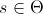. A standard way of doing such a test is to consider an rejection region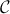. The test works as follows: consider a sample,

• if, then we accept• if, the we rejectFor instance, consider the case of a Bernoulli sample, with probability. The standard idea is to defineThe rejection region is then based on statistic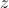,

• if, then we accept• if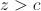, the we rejectwhere thresholdis taken so that the probability to make a first type error is(say 5%) using the Gaussian approximation for z. Here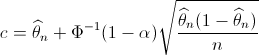Thus, the acceptation region is then the green area below, while the rejection region is the red one, for.Consider now the exact opposite test (with the same),versus. Here, we use the same statistics, and the test is

• if, then we accept• if, the we rejectwhere nowThus, now, the acceptation region is then the green area below, while the
rejection region is the red one.So if we summarize what we just said,

• in the region on the left below, both test agree that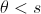• in the region on the right below, both test agree that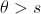• and in the region in blue, in the middle, the two tests disagree (one claims that, and the other one that)Here is the evolution of the region as a function of(the size of the sample) when the sample frequency is 20%. With a small sample size, we can hardly say anything.

`n=seq(1,100)p=0.2x1=p+qnorm(.95)*sqrt(p*(1-p)/n)x2=p+qnorm(.05)*sqrt(p*(1-p)/n)plot(n,x1,type="l",ylim=c(0,1))polygon(c(n,rev(n)),c(x1,rev(x2)),col="light blue",border=NA)lines(n,x1,lwd=2,col="red")lines(n,x2,lwd=2,col="red")`One might say that those bounds are based on a Gaussian approximation which is not correct whenis too small. So we can compute exact bounds,
`y1=qbinom(.95,size=n,prob=p)/ny2=qbinom(.05,size=n,prob=p)/npolygon(c(n,rev(n)),c(y1,rev(y2)),col="blue",border=NA)lines(n,y1,lwd=2,col="red")lines(n,y2,lwd=2,col="red")`

and we get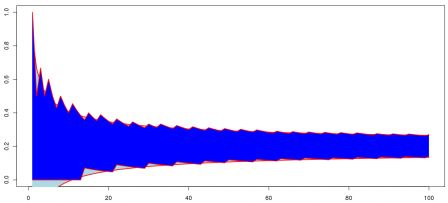This is what we can observe if we use R statistical procedures, either the asymptotic one,

`> prop.test(2,10,.5,alternative="less") 1-sample proportions test with continuity correction data:  2 out of 10, null probability 0.5X-squared = 2.5, df = 1, p-value = 0.05692alternative hypothesis: true p is less than 0.595 percent confidence interval:0.0000000 0.5100219sample estimates:p0.2 > prop.test(2,10,.5,alternative="greater") 1-sample proportions test with continuity correction data:  2 out of 10, null probability 0.5X-squared = 2.5, df = 1, p-value = 0.943alternative hypothesis: true p is greater than 0.595 percent confidence interval:0.04368507 1.00000000sample estimates:p0.2`

or a more accurate one

`> binom.test(2,10,.5,alternative="less") Exact binomial test data:  2 and 10number of successes = 2, number of trials = 10, p-value = 0.05469alternative hypothesis: true probability of success is less than 0.595 percent confidence interval:0.0000000 0.5069013sample estimates:probability of success0.2 > binom.test(2,10,.5,alternative="greater") Exact binomial test data:  2 and 10number of successes = 2, number of trials = 10, p-value = 0.9893alternative hypothesis: true probability of success is greater than 0.595 percent confidence interval:0.03677144 1.00000000sample estimates:probability of success0.2`Here, when the sample frequency is 20% andis equal to 10, we accept at the same time that theta is higher than 50% and lower than 50%.
And
obviously it is not only a theoretical problem: it has obviously some
strong implications. This morning, a good friend mentioned a post
published some months ago, online here, about discrimination, and the lack of women with academic positions in mathematics, in France. As claimed by the author of the postA Paris VI, meilleure université française selon son président, sur 11
postes de maitres de conférences, 5 filles classées premières. Il y a
donc des filles excellentes ? A Toulouse, sur 4 postes, 2 filles
premières. Parité parfaite. Mais à côté de cela, Bordeaux, 4 postes, 0
fille première. Littoral, 3 postes, 0 fille, Nice, 5 postes, 0 fille,
Rennes, 7 postes, 0 fille…
“.
Consider the latter one: in
Rennes, out of 7 people hired last year, no woman. So in some sense, it
looks obvious that there is some kind of discrimination ! Zero out of
seven ! Well, if we consider the fact that around 30% of PhD thesis in mathematics
were defended by women those years, we can also try to see is there if
no “positive discrimination“, i.e. test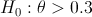where theta is the probability to hire a woman (just to be a little bit provocative).

`> prop.test(0,7,.3,alternative="less") 1-sample proportions test with continuity correction data:  0 out of 7, null probability 0.3X-squared = 1.7415, df = 1, p-value = 0.09347alternative hypothesis: true p is less than 0.395 percent confidence interval:0.0000000 0.3719021sample estimates:p0 Warning message:In prop.test(0, 7, 0.3, alternative = "less") :Chi-squared approximation may be incorrect> binom.test(0,7,.3,alternative="less") Exact binomial test data:  0 and 7number of successes = 0, number of trials = 7, p-value = 0.08235alternative hypothesis: true probability of success is less than 0.395 percent confidence interval:0.0000000 0.3481637sample estimates:probability of success0`

With no woman hired that year, we can still pretend that there was some kind of “positive discrimination“. An note that we do accept – with more confidence – the assumption of “positive discrimination” if we look at all universities together,

`> prop.test(5+2,11+4+4+3+5+7,.3,alternative="less") 1-sample proportions test with continuity correction data:  5 + 2 out of 11 + 4 + 4 + 3 + 5 + 7, null probability 0.3X-squared = 1.021, df = 1, p-value = 0.1561alternative hypothesis: true p is less than 0.395 percent confidence interval:0.0000000 0.3556254sample estimates:p0.2058824 > binom.test(5+2,11+4+4+3+5+7,.3,alternative="less") Exact binomial test data:  5 + 2 and 11 + 4 + 4 + 3 + 5 + 7number of successes = 7, number of trials = 34, p-value = 0.1558alternative hypothesis: true probability of success is less than 0.395 percent confidence interval:0.0000000 0.3521612sample estimates:probability of success0.2058824`

So obviously, with small sample, almost anything can be claimed !